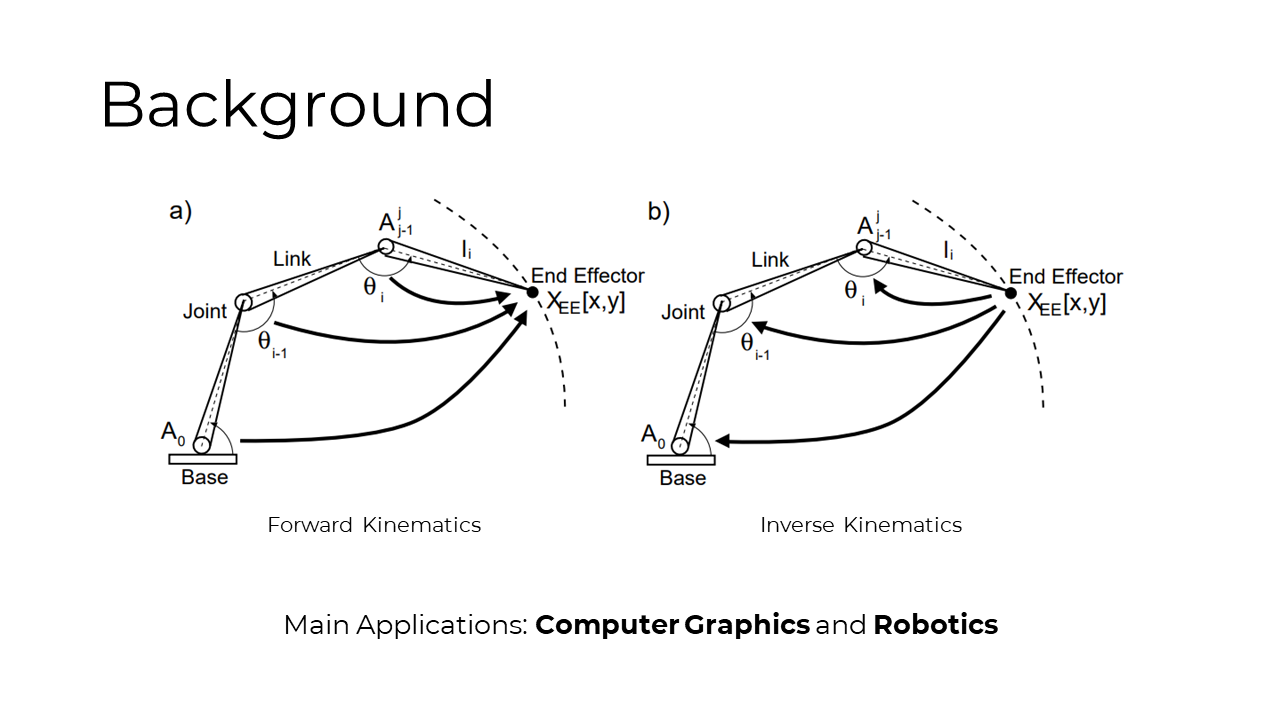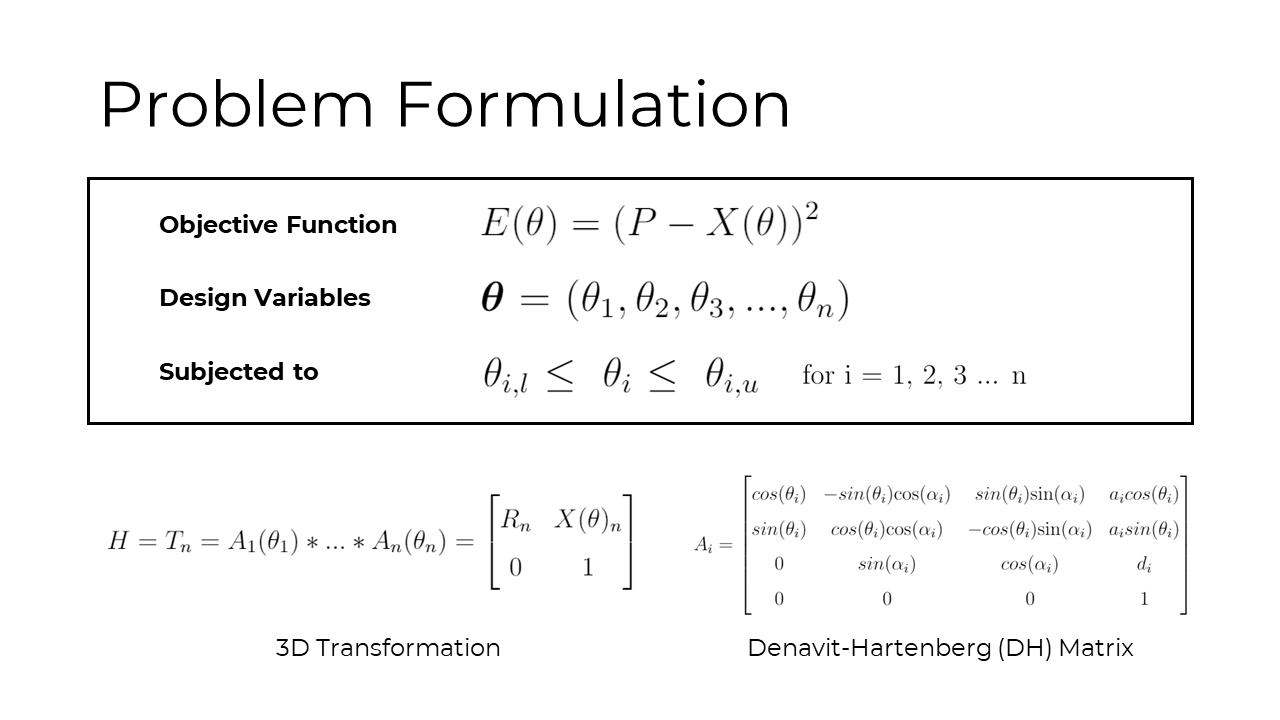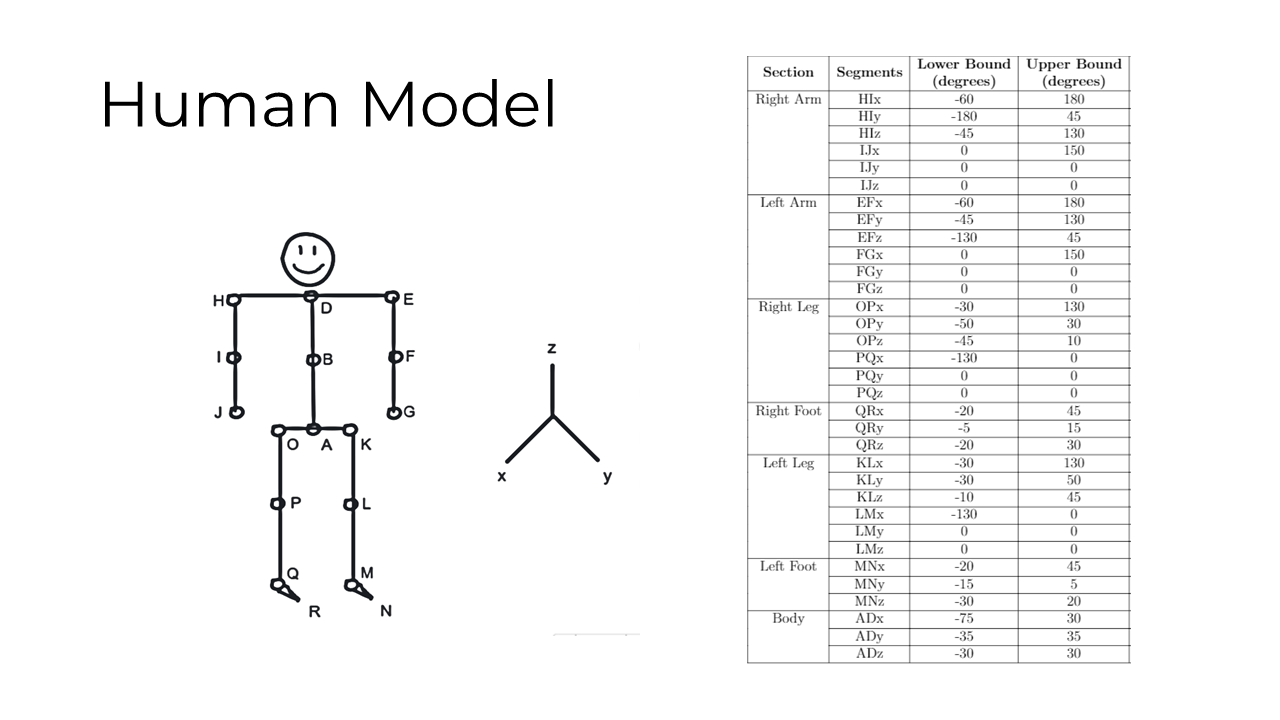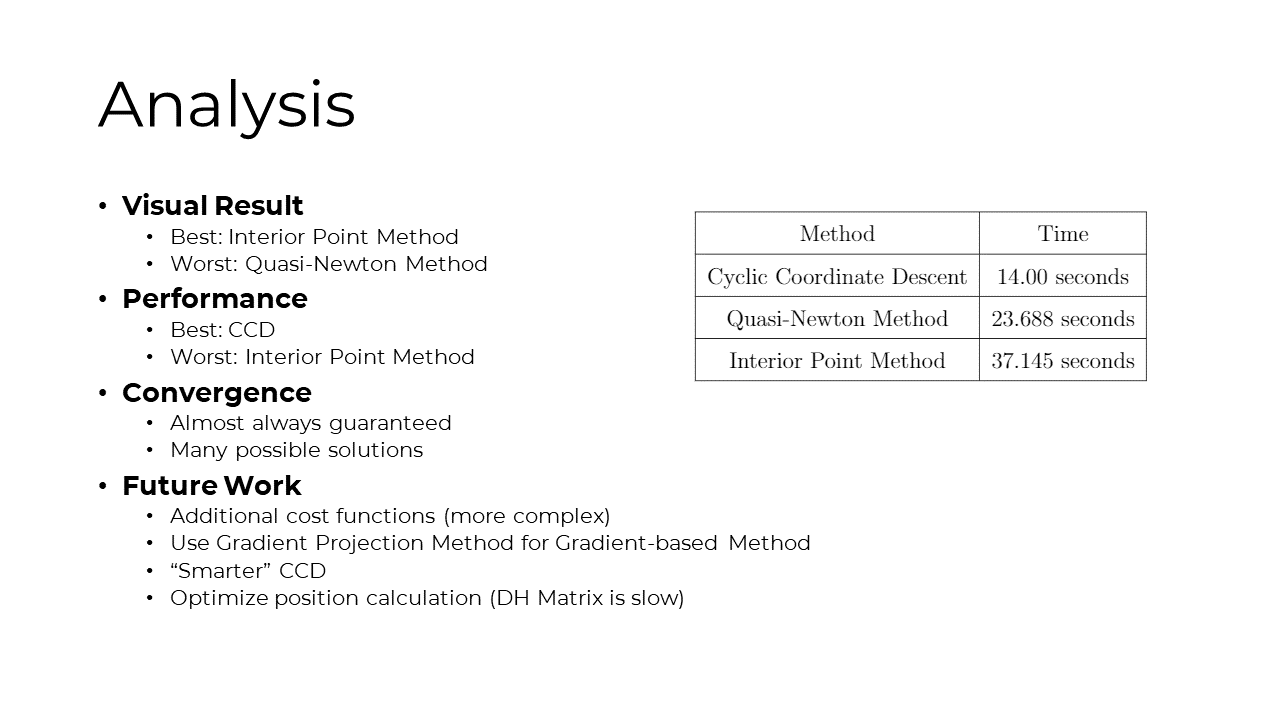## Inverse Kinematics as an Optimization Problem

Inverse kinematics has important applications in the field of computer graphics and robotics. The goal of this project is to understand IK as an optimization problem, and evaluate some of the numerical techniques used to solve the problem. IK system can be treated as an optimization problem by minimizing the distance error between the current and desired end effector positions. To calculate the current position, the Denavit-Hartenberg (DH) model is used for easier implementation (in MATLAB). A basic human rig is used in this project as the system to optimize for. Three optimization techniques were investigated, specifically the Interior Point method, the Quasi-Newton method, and the Cyclic Coordinate Descent method. Two animations were generated to analyze the visual result and computational performance of each method.### OverviewThe first method that was used to optimize the error function is MATLAB's built-in (fmincon) function, used to find the minimum of a constrained nonlinear multivariate function. The specific optimization algorithm that was used was the barrier or interior point method (IPM). Two animations were generated by animating the end effectors (red) of the IK chains.

The second method used is the gradient-based optimization method, using MATLAB's fminunc, which attempts to minimize an unconstrained function. Specifically, the Quasi-Newton Method was used with the Broyden-Fletcher-Goldfarb-Shanno (BFGS) algorithm for the search direction. In order to change the existing constrained problem into an unconstrained problem, a penalty function is introduced. The penalty function adds error penalties to the objective function if the angles are reaching beyond the allowed limits of joint rotation, thus deterring the algorithm from picking the position as an optimal position with minimal error. Such constraints are called soft-constraints because they do not render unfeasible limb postures from occurring, they only deter the algorithm from picking them.

The last technique used is Cyclic Coordinate Descent (CCD). CCD is a heuristic iterative approach where the transformation at each joint is optimized locally to have the end effector as close to the target as possible, while keeping other joint variables constant. This process, analogous to the Gauss-Seidel method, is continued until a solution converges. Advantages of CCD for inverse kinematic problems include being computationally fast, algorithmically simple and it is a straight-forward technique for generating solutions at high frame rates for animation. For these reasons, it is one of the most popular methods used to solve inverse kinematics systems. Since MATLAB does not have a built-in CCD function, a custom solver is implemented as part of the project following the work from this paper.Computationally, the interior point method is the slowest and fails to compute for large DOF. Quasi-Newton scales exponentially to the number of DOF, while CCD scales linearly. It is important to emphasize that none of the techniques has been optimized for IK system. The calculation of the current position using forward kinematics can also be drastically improved by using an alternative model to DH.